Chapter 1, Section 4## Solving Numeric Problems

Because measurement is such an important part of chemistry, most word problems in chemistry require some math. The techniques used in this book to solve numeric problems are conveniently organized into a three-step, problem-solving approach. This approach has been shown to be very helpful and effective. So we recommend that you follow this approach when working on numeric problems in this textbook. The steps for solving a numeric word problem are analyze, calculate, and evaluate. Figure 1.24 summarizes the three-step process and Sample Problem 1.1 shows how the steps work in a problem.

Figure 1.24 This flowchart summarizes the steps for solving a numeric problem. Predicting In which step do you make a plan for getting from what is known to what is unknown?

To solve a word problem, you must first determine where you are starting from (identify what is known) and where you are going (identify the unknown). What is known may be a measurement. Or it may be an equation that shows a relationship between measurements. If you expect the answer (the unknown) to be a number, you need to determine what units the answer should have before you do any calculations.

After you identify the known and the unknown, you need to make a plan for getting from the known to the unknown. Planning is at the heart of successful problem solving. As part of planning, you might draw a diagram that helps you visualize a relationship between the known and the unknown. You might need to use a table or graph to identify data or to identify a relationship between a known quantity and the unknown. You may need to select an equation that you can use to calculate the unknown.

If you make an effective plan, doing the calculations is usually the easiest part of the process. For some problems, you will have to convert a measurement from one unit to another. Or you may need to rearrange an equation before you can solve for an unknown. However, you will be taught these math skills as needed. There will also be reminders throughout the textbook to use the Math Handbook in Appendix C.

After you calculate an answer, you should evaluate it. Is the answer reasonable? Does it make sense? If not, reread the word problem. Did you copy the data correctly? Did you choose the right equations? It helps to round off the numbers and make an estimate of the answer. If the answer is much larger or much smaller than your estimate, check your calculations.

Check that your answer has the correct unit and the correct number of significant figures. You may need to use scientific notation in your answer. You will study significant figures and scientific notation in Chapter 3.

## Appendix A: Applications

Using a problem-solving strategy to solve number problems, learning outcomes.

• Apply the general problem-solving strategy to number problems
• Identify how many numbers you are solving for given a number problem
• Solve consecutive integer problems

Now we will translate and solve number problems. In number problems, you are given some clues about one or more numbers, and you use these clues to build an equation. Number problems don’t usually arise on an everyday basis, but they provide a good introduction to practicing the Problem-Solving Strategy. Remember to look for clue words such as difference , of , and and .

The difference of a number and six is $13$. Find the number.

https://ohm.lumenlearning.com/multiembedq.php?id=142763&theme=oea&iframe_resize_id=mom50

The sum of twice a number and seven is $15$. Find the number.

Show Solution

https://ohm.lumenlearning.com/multiembedq.php?id=142770&theme=oea&iframe_resize_id=mom60

Watch the following video to see another example of how to solve a number problem.

## Solving for Two or More Numbers

Some number word problems ask you to find two or more numbers. It may be tempting to name them all with different variables, but so far we have only solved equations with one variable. We will define the numbers in terms of the same variable. Be sure to read the problem carefully to discover how all the numbers relate to each other.

One number is five more than another. The sum of the numbers is twenty-one. Find the numbers.

https://ohm.lumenlearning.com/multiembedq.php?id=142775&theme=oea&iframe_resize_id=mom70

Watch the following video to see another example of how to find two numbers given the relationship between the two.

The sum of two numbers is negative fourteen. One number is four less than the other. Find the numbers.

https://ohm.lumenlearning.com/multiembedq.php?id=142806&theme=oea&iframe_resize_id=mom80

One number is ten more than twice another. Their sum is one. Find the numbers.

https://ohm.lumenlearning.com/multiembedq.php?id=142811&theme=oea&iframe_resize_id=mom90

## Solving for Consecutive Integers

Consecutive integers are integers that immediately follow each other. Some examples of consecutive integers are:

$\begin{array}{c}\phantom{\rule{0.2em}{0ex}}\\ \phantom{\rule{0.2em}{0ex}}\\ \phantom{\rule{0.2em}{0ex}}\\ \phantom{\rule{0.2em}{0ex}}\\ \hfill \text{…}1,2,3,4\text{,…}\hfill \end{array}$ $\text{…}-10,-9,-8,-7\text{,…}$ $\text{…}150,151,152,153\text{,…}$

Notice that each number is one more than the number preceding it. So if we define the first integer as $n$, the next consecutive integer is $n+1$. The one after that is one more than $n+1$, so it is $n+1+1$, or $n+2$.

$\begin{array}{cccc}n\hfill & & & \text{1st integer}\hfill \\ n+1\hfill & & & \text{2nd consecutive integer}\hfill \\ n+2\hfill & & & \text{3rd consecutive integer}\hfill \end{array}$

The sum of two consecutive integers is $47$. Find the numbers.

Find three consecutive integers whose sum is $42$.

Watch this video for another example of how to find three consecutive integers given their sum.

• Ex: Linear Equation Application with One Variable - Number Problem. Authored by : James Sousa (Mathispower4u.com). Located at : https://youtu.be/juslHscrh8s . License : CC BY: Attribution
• Ex: Write and Solve an Equation for Consecutive Natural Numbers with a Given Sum. Authored by : James Sousa (Mathispower4u.com). Located at : https://youtu.be/Bo67B0L9hGs . License : CC BY: Attribution
• Write and Solve a Linear Equations to Solve a Number Problem (1) Mathispower4u . Authored by : James Sousa (Mathispower4u.com) for Lumen Learning. Located at : https://youtu.be/izIIqOztUyI . License : CC BY: Attribution
• Question ID 142763, 142770, 142775, 142806, 142811, 142816, 142817. Authored by : Lumen Learning. License : CC BY: Attribution . License Terms : IMathAS Community License, CC-BY + GPL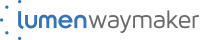## How to Solve Coding Problems with a Simple Four Step Method

I had fifteen minutes left, and I knew I was going to fail.

I had spent two months studying for my first technical interview.

I thought I was prepared, but as the interview came to a close, it hit me: I had no idea how to solve coding problems.

Of all the tutorials I had taken when I was learning to code, not one of them had included an approach to solving coding problems.

I had to find a method for problem-solving—my career as a developer depended on it.

I immediately began researching methods. And I found one. In fact, what I uncovered was an invaluable strategy. It was a time-tested four-step method that was somehow under the radar in the developer ecosystem.

In this article, I’ll go over this four-step problem-solving method that you can use to start confidently solving coding problems.

Solving coding problems is not only part of the developer job interview process—it’s what a developer does all day. After all, writing code is problem-solving.

## A method for solving problems

This method is from the book How to Solve It by George Pólya. It originally came out in 1945 and has sold over one million copies.

His problem-solving method has been used and taught by many programmers, from computer science professors (see Udacity’s Intro to CS course taught by professor David Evans) to modern web development teachers like Colt Steele.

Let’s walk through solving a simple coding problem using the four-step problem-solving method. This allows us to see the method in action as we learn it. We'll use JavaScript as our language of choice. Here’s the problem:

Create a function that adds together two numbers and returns that value. There are four steps to the problem-solving method:

• Understand the problem.
• Devise a plan.
• Carry out the plan.

Let’s get started with step one.

## Step 1: Understand the problem.

When given a coding problem in an interview, it’s tempting to rush into coding. This is hard to avoid, especially if you have a time limit.

However, try to resist this urge. Make sure you actually understand the problem before you get started with solving it.

Read through the problem. If you’re in an interview, you could read through the problem out loud if that helps you slow down.

As you read through the problem, clarify any part of it you do not understand. If you’re in an interview, you can do this by asking your interviewer questions about the problem description. If you’re on your own, think through and/or Google parts of the question you might not understand.

This first step is vital as we often don’t take the time to fully understand the problem. When you don’t fully understand the problem, you’ll have a much harder time solving it.

## What are the inputs?

What kinds of inputs will go into this problem? In this example, the inputs are the arguments that our function will take.

Just from reading the problem description so far, we know that the inputs will be numbers. But to be more specific about what the inputs will be, we can ask:

Will the inputs always be just two numbers? What should happen if our function receives as input three numbers?

Here we could ask the interviewer for clarification, or look at the problem description further.

The coding problem might have a note saying, “You should only ever expect two inputs into the function.” If so, you know how to proceed. You can get more specific, as you’ll likely realize that you need to ask more questions on what kinds of inputs you might be receiving.

Will the inputs always be numbers? What should our function do if we receive the inputs “a” and “b”? Clarify whether or not our function will always take in numbers.

Optionally, you could write down possible inputs in a code comment to get a sense of what they’ll look like:

//inputs: 2, 4

## What are the outputs?

What will this function return? In this case, the output will be one number that is the result of the two number inputs. Make sure you understand what your outputs will be.

## Create some examples.

Once you have a grasp of the problem and know the possible inputs and outputs, you can start working on some concrete examples.

Examples can also be used as sanity checks to test your eventual problem. Most code challenge editors that you’ll work in (whether it’s in an interview or just using a site like Codewars or HackerRank) have examples or test cases already written for you. Even so, writing out your own examples can help you cement your understanding of the problem.

What’s an example input? Example input might be:

What is the output to this? To write the example output, we can write:

This indicates that our function will take in an input of 2 and 3 and return 5 as its output.## Create complex examples.

By walking through more complex examples, you can take the time to look for edge cases you might need to account for.

For example, what should we do if our inputs are strings instead of numbers? What if we have as input two strings, for example, add('a', 'b')?

Your interviewer might possibly tell you to return an error message if there are any inputs that are not numbers. If so, you can add a code comment to handle this case if it helps you remember you need to do this.

Your interviewer might also tell you to assume that your inputs will always be numbers, in which case you don’t need to write any extra code to handle this particular input edge case.

If you don’t have an interviewer and you’re just solving this problem, the problem might say what happens when you enter invalid inputs.

For example, some problems will say, “If there are zero inputs, return undefined.” For cases like this, you can optionally write a comment.

// check if there are no inputs.

// If no inputs, return undefined.

For our purposes, we’ll assume that our inputs will always be numbers. But generally, it’s good to think about edge cases.

Computer science professor Evans says to write what developers call defensive code. Think about what could go wrong and how your code could defend against possible errors.

Before we move on to step 2, let’s summarize step 1, understand the problem:

-What are the inputs?

-What are the outputs?

Create simple examples, then create more complex ones.

## 2. Devise a plan for solving the problem.

Next, devise a plan for how you’ll solve the problem. As you devise a plan, write it out in pseudocode.

Pseudocode is a plain language description of the steps in an algorithm. In other words, your pseudocode is your step-by-step plan for how to solve the problem.

Write out the steps you need to take to solve the problem. For a more complicated problem, you’d have more steps. For this problem, you could write:

// Create a sum variable.

Add the first input to the second input using the addition operator .

// Store value of both inputs into sum variable.

// Return as output the sum variable. Now you have your step-by-step plan to solve the problem. For more complex problems, professor Evans notes, “Consider systematically how a human solves the problem.” That is, forget about how your code might solve the problem for a moment, and think about how you would solve it as a human. This can help you see the steps more clearly.

## 3. Carry out the plan (Solve the problem!)The next step in the problem-solving strategy is to solve the problem. Using your pseudocode as your guide, write out your actual code.

Professor Evans suggests focusing on a simple, mechanical solution. The easier and simpler your solution is, the more likely you can program it correctly.

Taking our pseudocode, we could now write this:

Professor Evans adds, remember not to prematurely optimize. That is, you might be tempted to start saying, “Wait, I’m doing this and it’s going to be inefficient code!”

First, just get out your simple, mechanical solution.

What if you can’t solve the entire problem? What if there's a part of it you still don't know how to solve?

Colt Steele gives great advice here: If you can’t solve part of the problem, ignore that hard part that’s tripping you up. Instead, focus on everything else that you can start writing.

Temporarily ignore that difficult part of the problem you don’t quite understand and write out the other parts. Once this is done, come back to the harder part.

This allows you to get at least some of the problem finished. And often, you’ll realize how to tackle that harder part of the problem once you come back to it.

## Step 4: Look back over what you've done.

Once your solution is working, take the time to reflect on it and figure out how to make improvements. This might be the time you refactor your solution into a more efficient one.

As you look at your work, here are some questions Colt Steele suggests you ask yourself to figure out how you can improve your solution:

• Can you derive the result differently? What other approaches are there that are viable?
• Can you understand it at a glance? Does it make sense?
• Can you use the result or method for some other problem?
• Can you improve the performance of your solution?
• Can you think of other ways to refactor?
• How have other people solved this problem?

One way we might refactor our problem to make our code more concise: removing our variable and using an implicit return:

With step 4, your problem might never feel finished. Even great developers still write code that they later look at and want to change. These are guiding questions that can help you.

If you still have time in an interview, you can go through this step and make your solution better. If you are coding on your own, take the time to go over these steps.

When I’m practicing coding on my own, I almost always look at the solutions out there that are more elegant or effective than what I’ve come up with.

## Wrapping Up

In this post, we’ve gone over the four-step problem-solving strategy for solving coding problems.

Let's review them here:

• Step 1: understand the problem.
• Step 2: create a step-by-step plan for how you’ll solve it .
• Step 3: carry out the plan and write the actual code.
• Step 4: look back and possibly refactor your solution if it could be better.

Practicing this problem-solving method has immensely helped me in my technical interviews and in my job as a developer. If you don't feel confident when it comes to solving coding problems, just remember that problem-solving is a skill that anyone can get better at with time and practice.

## If you enjoyed this post, join my coding club , where we tackle coding challenges together every Sunday and support each other as we learn new technologies.

If you have feedback or questions on this post, feel free to tweet me @madisonkanna ..

If you read this far, tweet to the author to show them you care. Tweet a thanks

Learn to code for free. freeCodeCamp's open source curriculum has helped more than 40,000 people get jobs as developers. Get started

• What is Chemical and Biological Engineering?
• Engineering problem solving
• Error and uncertainty
• Process variables
• Process Fundamentals
• Material Balances
• Reacting systems
• Reaction kinetics
• Bioreactors
• Reactor design
• Fluids and fluid flow
• Mass transfer
• Energy balances
• Heat transfer
• Heat exchangers
• Mechanical energy balances
• Process safety
• Engineering ethics
• Sustainability
• Engineering in a global context
• How ‘good’ a solution do you need
• Steps in solving well-defined engineering process problems
• « What is Chemi...
• Teamwork »

## Engineering Problem Solving ¶

Some problems are so complex that you have to be highly intelligent and well-informed just to be undecided about them. —Laurence J. Peter

## Steps in solving ‘real world’ engineering problems ¶

The following are the steps as enumerated in your textbook:

Collaboratively define the problem

List possible solutions

Evaluate and rank the possible solutions

Develop a detailed plan for the most attractive solution(s)

Re-evaluate the plan to check desirability

Implement the plan

Check the results

A critical part of the analysis process is the ‘last’ step: checking and verifying the results.

Depending on the circumstances, errors in an analysis, procedure, or implementation can have significant, adverse consequences (NASA Mars orbiter crash, Bhopal chemical leak tragedy, Hubble telescope vision issue, Y2K fiasco, BP oil rig blowout, …).

In a practical sense, these checks must be part of a comprehensive risk management strategy.

My experience with problem solving in industry was pretty close to this, though encumbered by numerous business practices (e.g., ‘go/no-go’ tollgates, complex approval processes and procedures).

In addition, solving problems in the ‘real world’ requires a multidisciplinary effort, involving people with various expertise: engineering, manufacturing, supply chain, legal, marketing, product service and warranty, …

Exercise: Problem solving

Step 3 above refers to ranking of alternatives.

Think of an existing product of interest.

What do you think was ranked highest when the product was developed?

Consider what would have happened if a different ranking was used. What would have changed about the product?

Brainstorm ideas with the students around you.

## Defining problems collaboratively ¶

Especially in light of global engineering , we need to consider different perspectives as we define our problem. Let’s break the procedure down into steps:

Identify each perspective that is involved in the decision you face. Remember that problems often mean different things in different perspectives. Relevant differences might include national expectations, organizational positions, disciplines, career trajectories, etc. Consider using the mnemonic device “Location, Knowledge, and Desire.”

Location : Who is defining the problem? Where are they located or how are they positioned? How do they get in their positions? Do you know anything about the history of their positions, and what led to the particular configuration of positions you have today on the job? Where are the key boundaries among different types of groups, and where are the alliances?

Knowledge : What forms of knowledge do the representatives of each perspective have? How do they understand the problem at hand? What are their assumptions? From what sources did they gain their knowledge? How did their knowledge evolve?

Desire : What do the proponents of each perspective want? What are their objectives? How do these desires develop? Where are they trying to go? Learn what you can about the history of the issue at hand. Who might have gained or lost ground in previous encounters? How does each perspective view itself at present in relation to those it envisions as relevant to its future?

As formal problem definitions emerge, ask “Whose definition is this?” Remember that “defining the problem clearly” may very well assert one perspective at the expense of others. Once we think about problem solving in relation to people, we can begin to see that the very act of drawing a boundary around a problem has non-technical, or political dimensions, depending on who controls the definition, because someone gains a little power and someone loses a little power.

Map what alternative problem definitions mean to different participants. More than likely you will best understand problem definitions that fit your perspective. But ask “Does it fit other perspectives as well?” Look at those who hold Perspective A. Does your definition fit their location, their knowledge, and their desires? Now turn to those who hold Perspective B. Does your definition fit their location, knowledge, and desires? Completing this step is difficult because it requires stepping outside of one’s own perspective and attempting to understand the problem in terms of different perspectives.

To the extent you encounter disagreement or conclude that the achievement of it is insufficient, begin asking yourself the following: How might I adapt my problem definition to take account of other perspectives out there? Is there some way of accommodating myself to other perspectives rather than just demanding that the others simply recognize the inherent value and rationality of mine? Is there room for compromise among contrasting perspectives?

## How ‘good’ a solution do you need ¶

There is also an important aspect of real-world problem solving that is rarely articulated and that is the idea that the ‘quality’ of the analysis and the resources expended should be dependent on the context.

This is difficult to assess without some experience in the particular environment.

How ‘Good’ a Solution Do You Need?

Some rough examples:

10 second answer (answering a question at a meeting in front of your manager or vice president)

10 day answer (assembling information as part of a trouble-shooting team)

10 month answer (putting together a comprehensive portfolio of information as part of the design for a new \$200,000,000 chemical plant)

## Steps in solving well-defined engineering process problems ¶

These steps could correspond to those needed to solve a textbook problem or part of the analysis for item 4 above.

Draw a diagram if one is not already available.

Write all known quantities (flow rates, densities, etc.) in the appropriate locations on the diagram. If symbols are used to designate known quantities, include those symbols on the diagram.

Identify and assign symbols to all unknown quantities and write them in the appropriate locations on the diagram.

Select a basis if needed: If no flow rates are known, assume a convenient value for one of the flow rates as a basis of calculation (e.g, $$\SI{100}{lbmol/s}$$ , $$\SI{100}{kg/hr}$$ , etc.).

Determine the appropriate set of equations needed to solve for the unknown quantities. In order to solve for all of the unknowns, the number of equations must equal the number of unknowns. The steps below can be used to obtain the desired set of equations.

Construct the material balance equation(s):

Replace remaining terms with more convenient forms (because of given information or selected symbols)

Construct equations to express other known relationships between variables (Remember, the total number of equations must equal the number of unknowns).

Solve algebraically and then numerically: Solve algebraically for the desired parameters and then determine their values.

A glaring omission in this procedure is a check of the answer.

Exercise: Checking results

This may be relatively easy for a homework problem, but what about your analysis for an ill-defined ‘real-world’ problem?#### IMAGES

1. PPT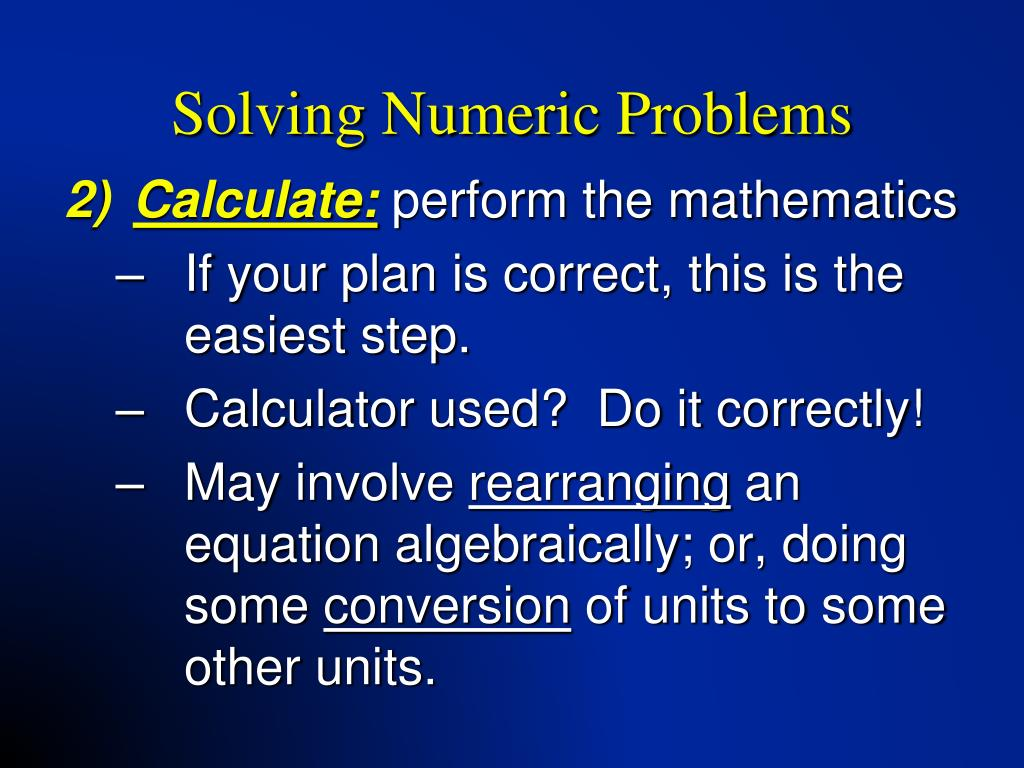2. PPT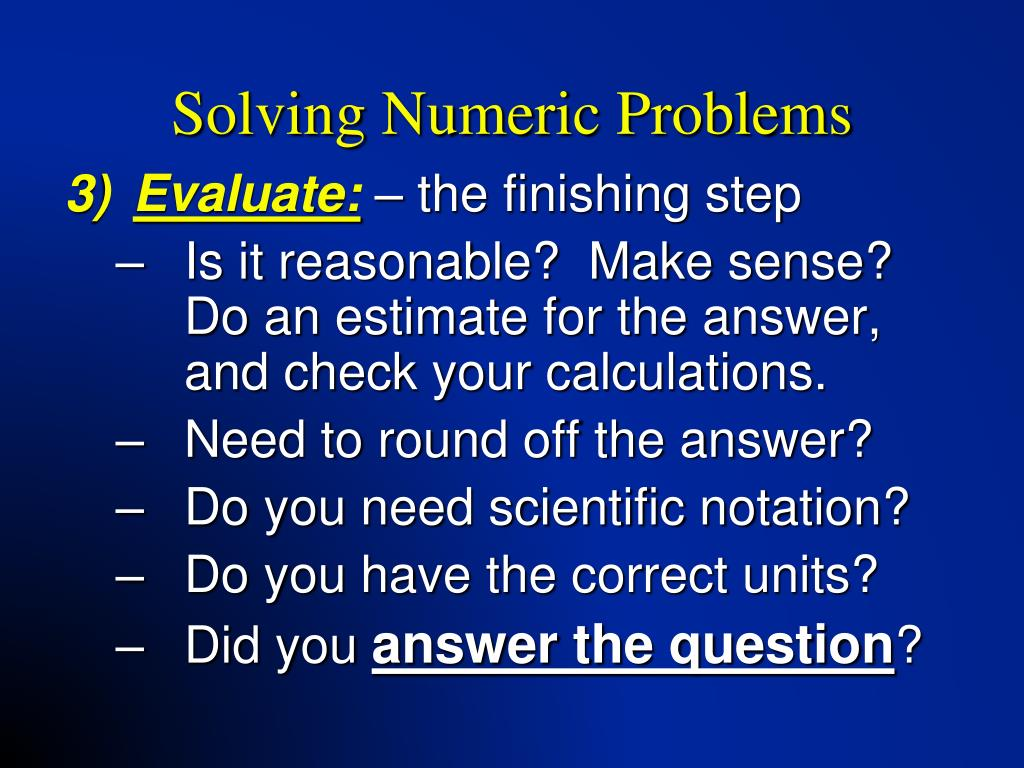3. 7 Effective Problem Solving Steps in the Workplace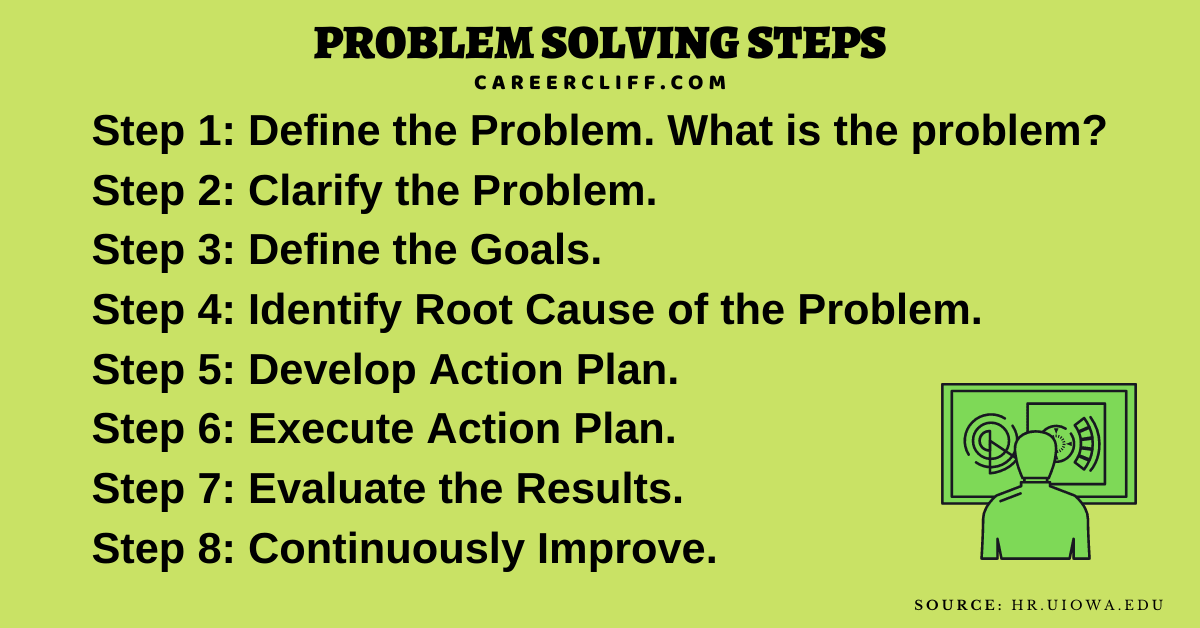4. Pin by Erin Haack on Math5. PPT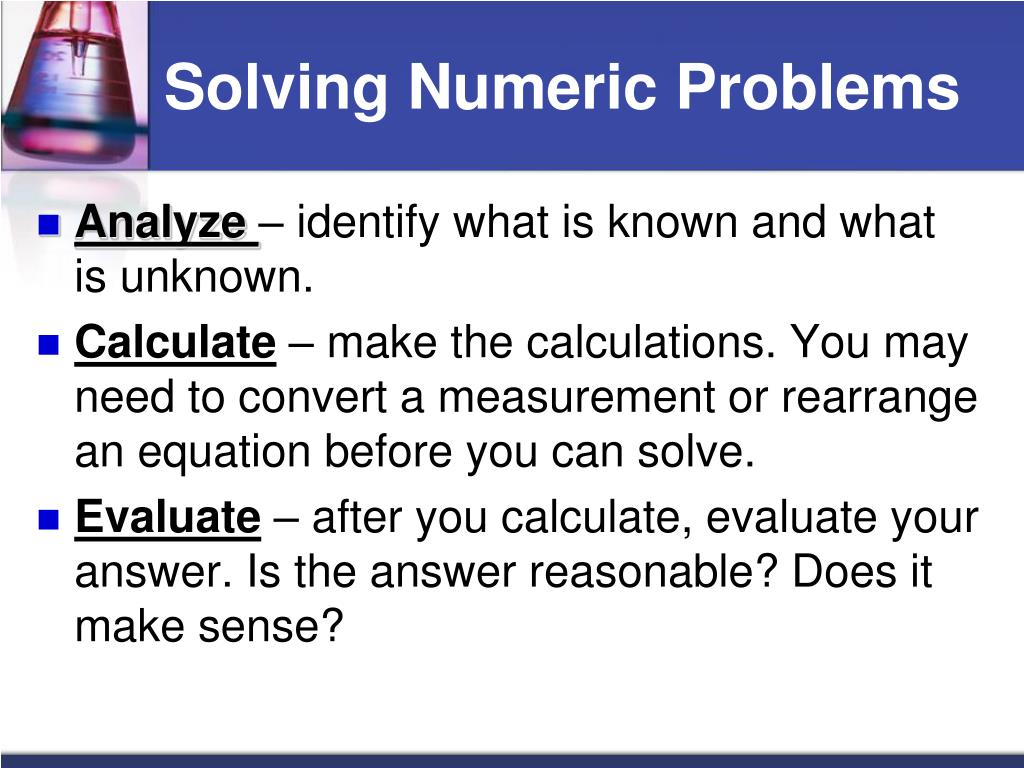6. Problem Solving: Using Charts II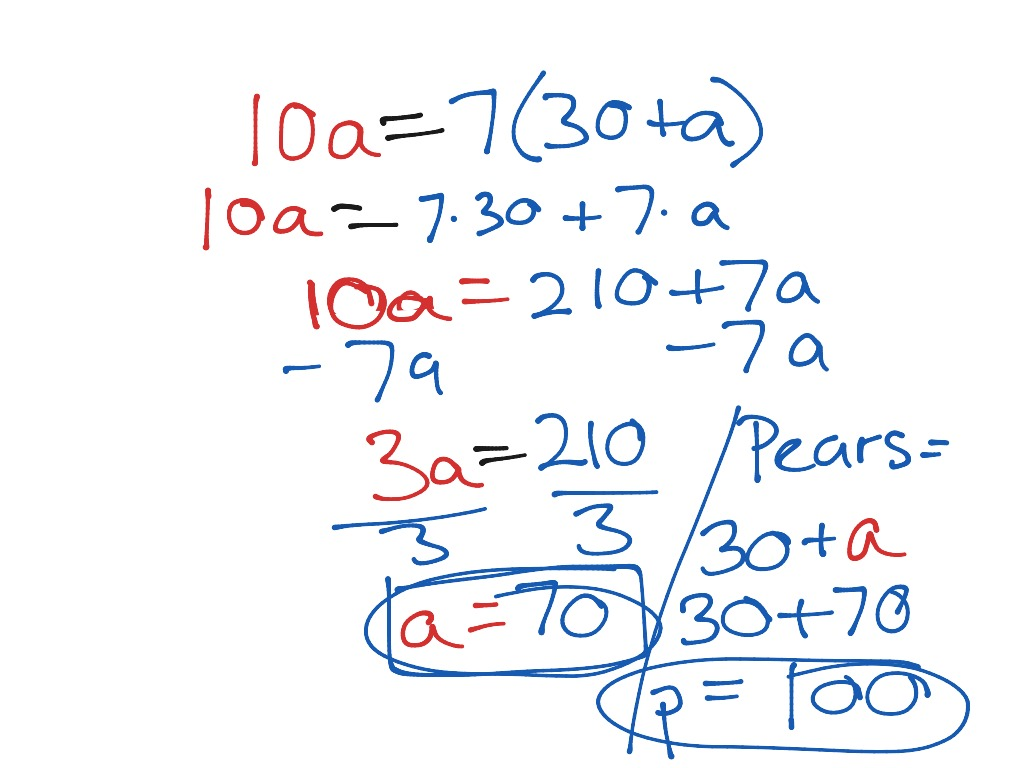#### VIDEO

1. how to solve maths problems quickly

2. how to solve problems

3. Solve the problem and find 🍄×🍏+🍉=??

4. Last step 💚🥰

5. numerical problem

6. How To Solve Any Problem BEST METHOD

1. Solving Bosch Dishwasher Problems: A Step-by-Step Guide

Having a dishwasher is a great convenience, but when it stops working properly, it can be a major inconvenience. Bosch dishwashers are known for their reliability and durability, but they can still experience problems from time to time.

2. What Is the Six-Step Decision Making Process?

The six-step decision-making process is called rational decision-making, and it utilizes analysis and facts to make a decision. To complete the first step in the rational decision-making process, one must identify the problem and then state...

3. Common Problems Solved by Dialing Comcast’s Toll-Free Number

Comcast is one of the largest telecommunications companies in the United States, providing a wide range of services including cable television, internet, and phone services. With millions of customers nationwide, it’s no surprise that occas...

4. the step that usually comes last in solving numeric problems is to

Answer: The step that usually comes last in solving numeric problem is to evaluate. Explanation: First step is Analyze .

5. Solving Numeric Problems

The steps for solving a numeric word problem are analyze, calculate, and evaluate. Figure 1.24 summarizes the three-step process and Sample Problem 1.1 shows

6. 1.4: Problem Solving in Chemistry Flashcards

The steps for solving a numeric word problem are analyze, calculate, and evaluate. What are the steps of solving nonnumeric problems?

7. Problem Solving in Chemistry Solving Numeric Problems Analyze

The steps for solving a numeric word problem are analyze, calculate, and evaluate. 1.4. Slide. 8 of 27. ©. Copyright Pearson Prentice Hall. >.

8. Chemistry Chapter 1 Flashcards

The step that usually comes last in numeric problems is to... Evaluate. How many steps are involved in solving numeric problems? Three steps are involved.

9. Using a Problem-Solving Strategy to Solve Number Problems

Apply the general problem-solving strategy to number problems · Identify how many numbers you are solving for given a number problem · Solve consecutive integer

10. HOW TO SOLVE ANY NUMERICAL PROBLEMS IN PHYSICS

HOW TO SOLVE ANY NUMERICAL PROBLEMS IN PHYSICS | CHEMISTRY.. BEST WAY TO SOLVE ANY PHYSICS NUMERICAL.. EASY WAY TO SOLVE ANY PHYSICS PROBLEM

11. Steps for Solving Number Stories

Hello Friends, Here are the steps that you can apply to solve any kind of Word Problem. We have taken a word problem to explain how to

12. How to Solve Coding Problems with a Simple Four Step Method

Create a function that adds together two numbers and returns that value. There are four steps to the problem-solving method: Understand the

13. Engineering Problem Solving

A critical part of the analysis process is the 'last' step: checking and verifying the results. Depending on the circumstances, errors in an analysis

14. How to improve my mathematical and numerical problem solving skill

Last summer, I started preparing for Software Engineering internship applications. I solved a few hundred questions on HackerRank and, in a few weeks, I managed